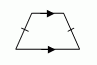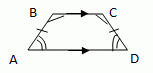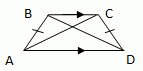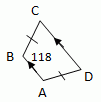kwizNET Subscribers, please login to turn off the Ads!
 Email us to get an instant 20% discount on highly effective K-12 Math & English kwizNET Programs!

#### Online Quiz (WorksheetABCD)

Questions Per Quiz = 2 4 6 8 10

### Geometry3.12 Trapezoid

 Trapezoid Is a quadrilateral with exactly one pair of parallel sides. The parallel sides are the bases A trapezoid has two pairs of base angles. For example in the trapezoid ABCD,�A and �B are one pair of base angles, and �C and �D are the second pair. The non parallel sides are the legs of the trapezoid. Isosceles Trapezoid: If the legs of the trapezoid are congruent then the trapezoid is an Isosceles trapezoid.THEOREMS: If a trapezoid is isosceles, then each pair of base angles is congruent. If trapezoid ABCD is isosceles, then �A @ �D and �B @ �C.If a trapezoid has a pair of congruent base angles, then it is an isosceles trapezoid ABCD is isosceles. If �A @ �D and �B @ �C. then ABCD is isosceles.A trapezoid is isosceles if and only if its diagonals are congruent. Trapezoid ABCD is isosceles if and only if AC @ BD.Midsegment Theorem for Trapezoids: The midsegment of a trapezoid is parallel to each base and its length is one half the sum of the lengths of the bases. If MN is the midsegment of trapezoid ABCD, then MN // AB, MN // DC, and MN = 1/2(AB+CD)Directions: 1) For the trapezoid ABCD shown below find the measures of angle A, angle C and angle D.2) Answer the following questions and write at least 5 examples of your own.
 Q 1: ABCD is a trapezoid. Using the mid segment theorem find the value of x.Answer: Q 2: A trapezoid has two pairs of _____ angles.baseleg Q 3: If the legs of a trapezoid are congruent, then the trapezoid is an ________________.isosceles trapezoidscalene trapezoidequilateral trapezoid Q 4: ABCD is a trapezoid. Measure of angle B is 1180. Find the measure of angle A.Answer: Question 5: This question is available to subscribers only! Question 6: This question is available to subscribers only!

#### Subscription to kwizNET Learning System offers the following benefits:

• Unrestricted access to grade appropriate lessons, quizzes, & printable worksheets
• Instant scoring of online quizzes
• Progress tracking and award certificates to keep your student motivated
• Unlimited practice with auto-generated 'WIZ MATH' quizzes
• Child-friendly website with no advertisements
• Choice of Math, English, Science, & Social Studies Curriculums
• Excellent value for K-12 and ACT, SAT, & TOEFL Test Preparation
• Get discount offers by sending an email to discounts@kwiznet.com

 Quiz Timer